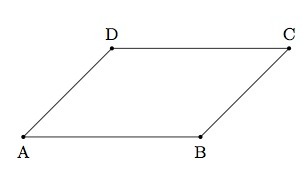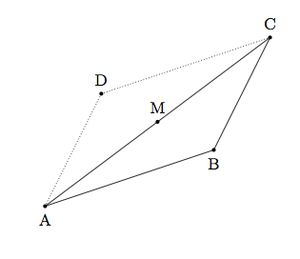# Congruence of parallelograms

Alignments to Content Standards: G-CO.C.11 G-SRT.B.5

Rhianna has learned the SSS and SAS congruence tests for triangles and she wonders if these tests might work for parallelograms.

1. Suppose $ABCD$ and $EFGH$ are two parallelograms all of whose corresponding sides are congruent, that is $|AB| = |EF|, |BC| = |FG|, |CD| = |GH|,$ and $|DA| = |HE|$. Is it always true that $ABCD$ is congruent to $EFGH$?
2. Suppose $ABCD$ and $EFGH$ are two parallelograms with a pair of congruent corresponding sides, $|AB| = |EF|$ and $|BC| = |FG|$. Suppose also that the included angles are congruent,
$m(\angle ABC) = m(\angle EFG)$. Are $ABCD$ and $EFGH$ congruent?

## IM Commentary

Triangle congruence criteria have been part of the geometry curriculum for centuries. For quadrilaterals, on the other hand, these nice tests seem to be lacking. This task addresses this issue for a specific class of quadrilaterals, namely parallelograms. This task is ideal for hands-on work or work with a computer to help visualize the possibilities. It turns out that knowing all four sides of two quadrilaterals are congruent is not enough to conclude that the quadrilaterals are congruent. Unlike with triangles, some information about angles is needed in order to conclude that two quadrilaterals are congruent.

If manipulatives are available, it would be valuable to use toothpicks for example to see that with three of them only one triangular shape is possible, namely an equilateral triangle. For quadrilaterals, on the other hand, four toothpicks can be put together to make any of the rhombuses with that side length. Just as with a triangle it takes three pieces of information (ASA, SAS, or SSS) to determine a shape, so with a quadrilateral we would expect to require four pieces of information. Four sides is not enough, but what about other combinations such as SASA? An interesting extension of this activity would be to have students make and verify conjectures about how much information is needed to determine if two quadrilaterals are congruent. For example, for squares one side is enough, for rectangles two adjacent sides are sufficient. What about for arbitrary quadrilaterals?

This task would be ideally suited for group work since it is open ended and calls for experimentation. Thus it provides a good opportunity for students to engage in MP3 ''Construct Viable Arguments and Critique the Reasoning of Others.'' Also as noted above, students working on this task have multiple opportunities to engage in MP5 ''Use Appropriate Tools Strategically'' as they can use manipulatives or computer software to experiment with constructing different parallelograms.

## Solutions

1. We begin by drawing or building a parallelogram. The opposite sides of a parallelogram are congruent so we will need two pairs of congruent segments:Now if we imagine leaving $\overline{AB}$ fixed and ''pushing down'' on side $\overline{CD}$ so that these two sides become closer while side $\overline{AD}$ and $\overline{BC}$ rotate clockwise we get a new parallelogram:Parallelograms $ABCD$ and $EFGH$ have four congruent sides but they are not congruent since they have different angles (and also different area). Also interesting in this case is that to the eye side $\overline{EH}$ does not appear to the eye to be congruent to side $\overline{AD}$: this could be an optical illusion or it could be that the eye is distracted by the difference in area.

2. We know from the SAS triangle congruence test that $\triangle ABC$ is congruent to $\triangle EFG$. In order to see what happens with the parallelograms $ABCD$ and $EFGH$ we focus first on $ABCD$. Note that the vertex $D$ is obtained by rotating $B$ 180 degrees about the midpoint $M$ of $\overline{AC}$. This is pictured below with the image of $B$ labeled $D$:In other words the parallelogram $ABCD$ is obtained by adjoining to $\triangle ABC$ a second triangle, $\triangle CDA$, which is congruent to $\triangle ABC$. The same is true of parallelogram $EFGH$ (which is obtained by adjoining $\triangle GHE$ to $\triangle EFG$) and since $\triangle ABC$ is congruent to $\triangle EFG$ (and $\triangle CDA$ is congruent to $\triangle GHE$) we can conclude that parallelogram $ABCD$ is congruent to parallelogram $EFGH$.

Solution: 2 Looking at a special case for part (a): the rhombus

1. We can look at what happens in the special case where all 4 sides of both $ABCD$ and $EFGH$ are congruent to one another. The same thing goes wrong in this case but it is interesting to consider and provides an opportunity to study some of the special types of parallelograms.

One way all sides of the two parallelograms could be congruent would be if $ABCD$ and $EFGH$ are squares with the same side length: in this case they would be congruent. More generally, a quadrilateral with 4 congruent sides is a rhombus. Once the side length is fixed, there are many possible rhombuses with the given side length as the angles can be varied as depicted in the pictures below:The first rhombus above is a square while the second one has angles of 60 and 120 degrees. Note that a rhombus is determined by one side length and a single angle: the given side length determines all four side lengths and opposite angles are congruent while adjacent angles are supplementary.